## Cloudknot:

### A Python library to run your existing code on AWS Batch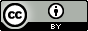## Ariel• Senior Data Scientist, UW eScience Institute
• Open source software for neuroscience
• Software and Data Carpentry Instructor and Instructor Trainer
• Chair of eScience working group on reproducibility and open science• PhD Candidate, UW Physics
• Nuclear theory: quantum Monte Carlo studies of neutron matter
• Neuroscience: visualization and machine learning tools for neuroimaging data
• Software and Data Carpentry Instructor

## We use Python

• Post-processing of QMC results
• Computational neuroanatomy, DIPY
• White matter tractometry, pyAFQ

## We love working in our Python environment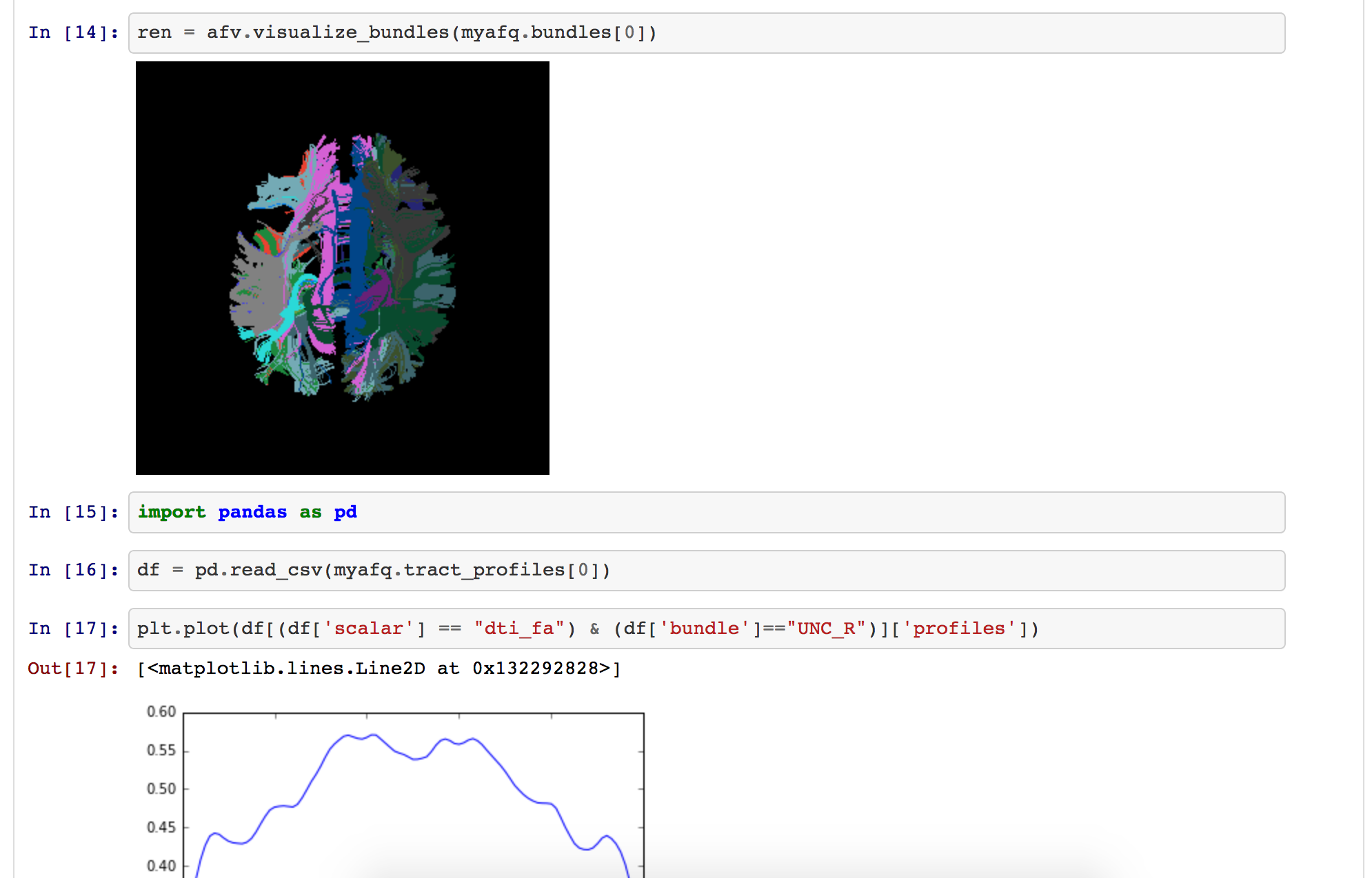Diffusion MRI analysis using pyAFQ in a Jupyter notebook

## We also like to use AWS

Pros:

• Linear scalability
• Elasticity
• Ability to handle large datasets

Cons:

• Learning curve
• Difficult to choose instances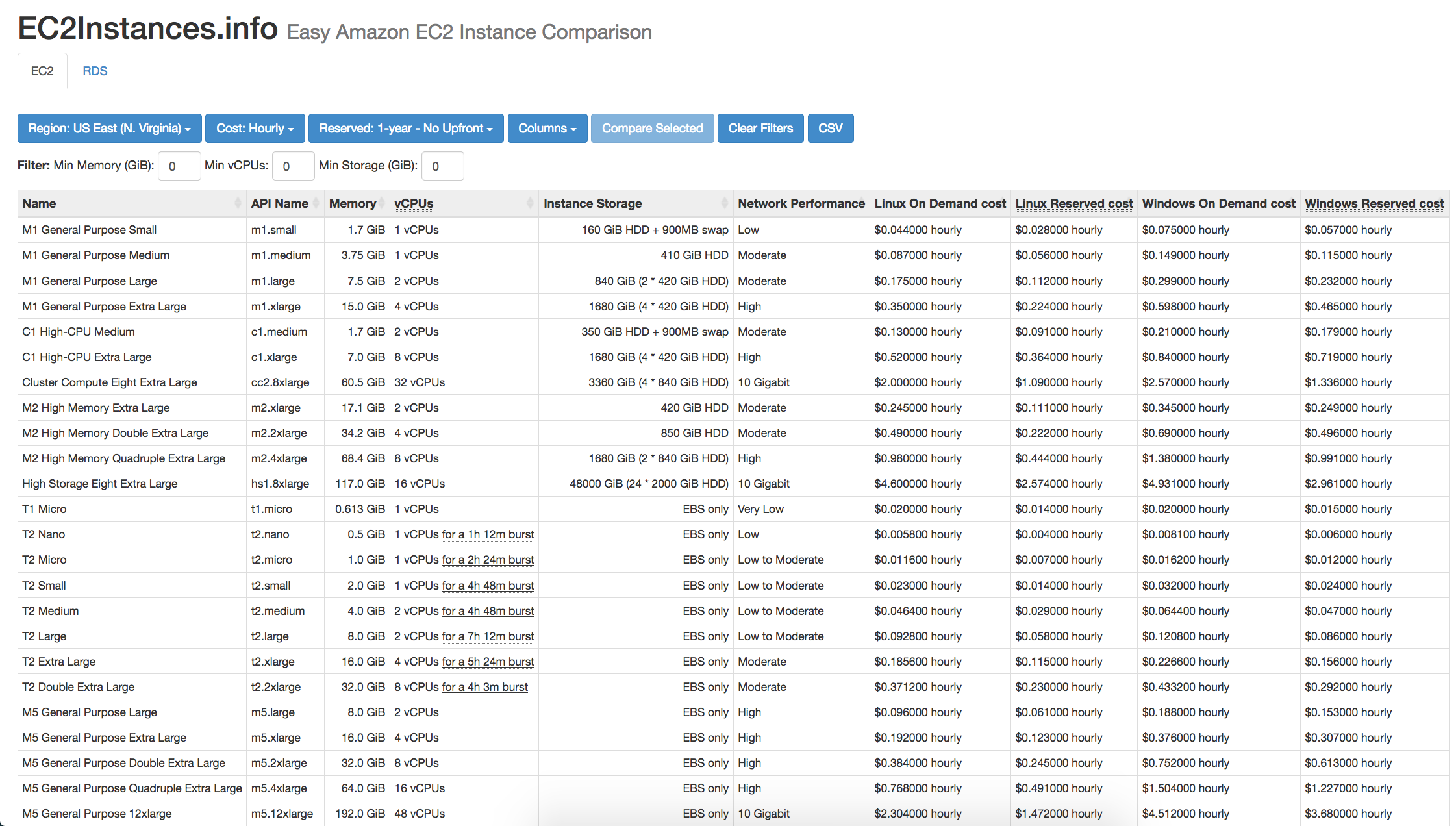ec2instances.info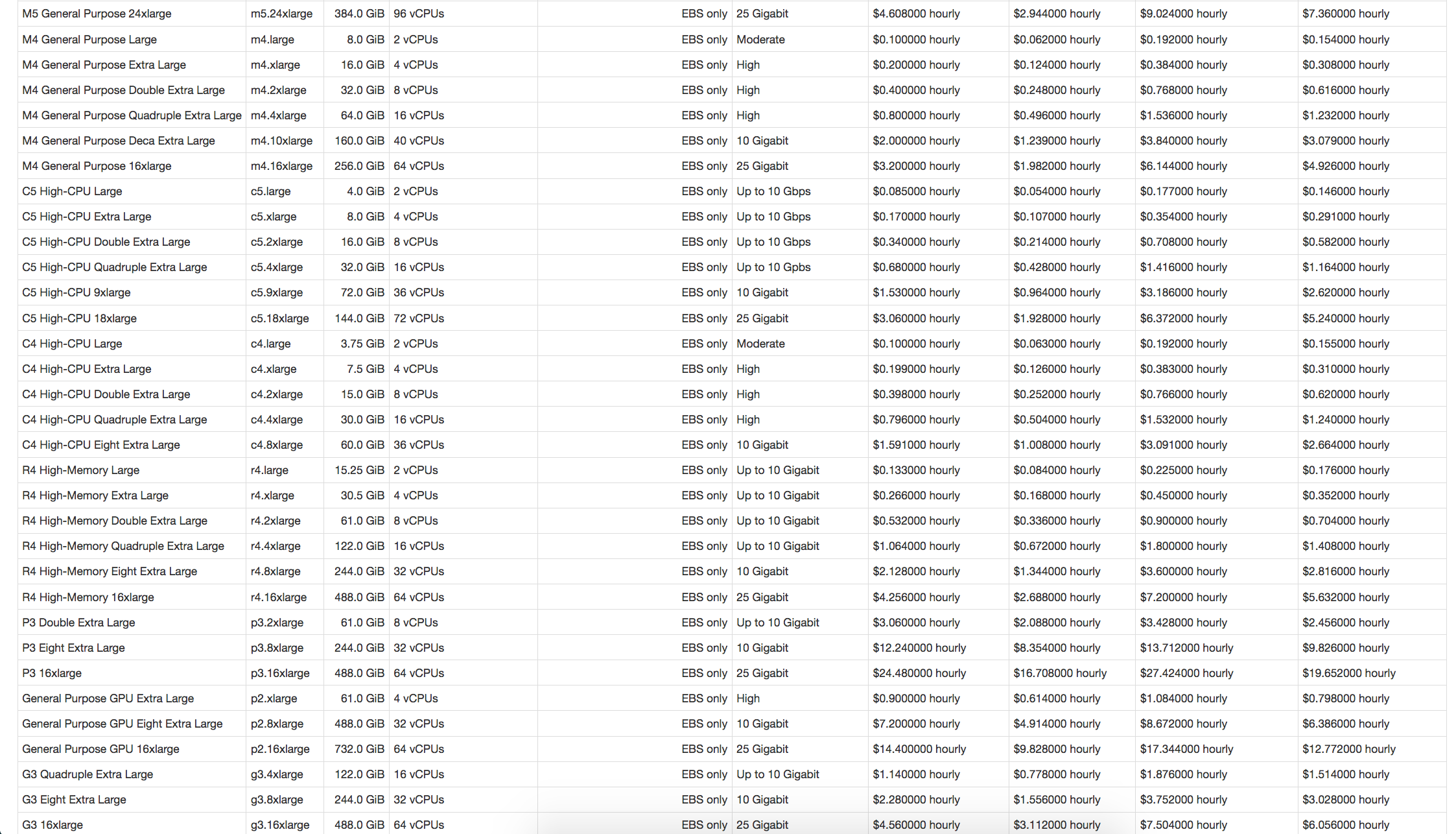ec2instances.info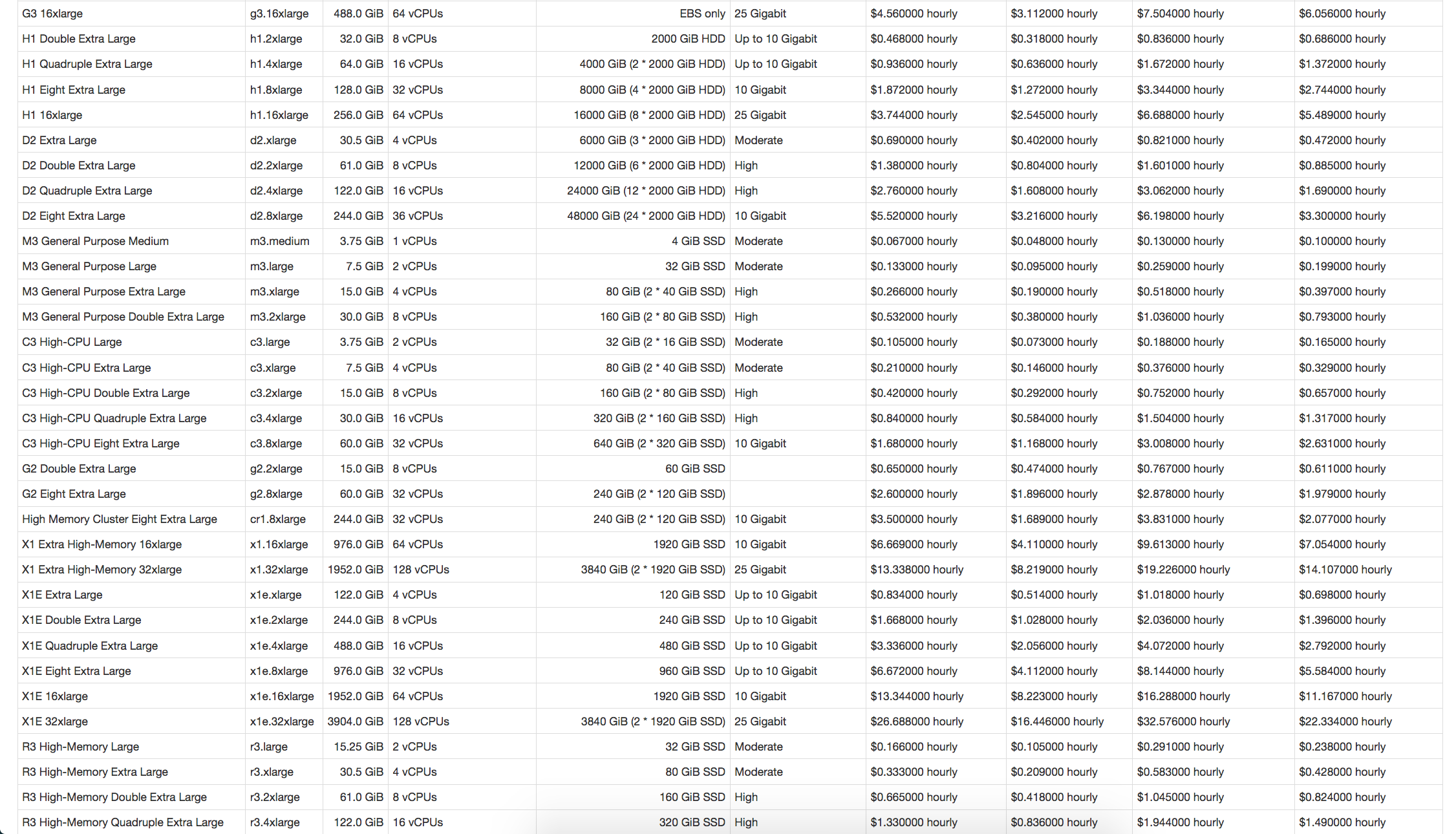ec2instances.info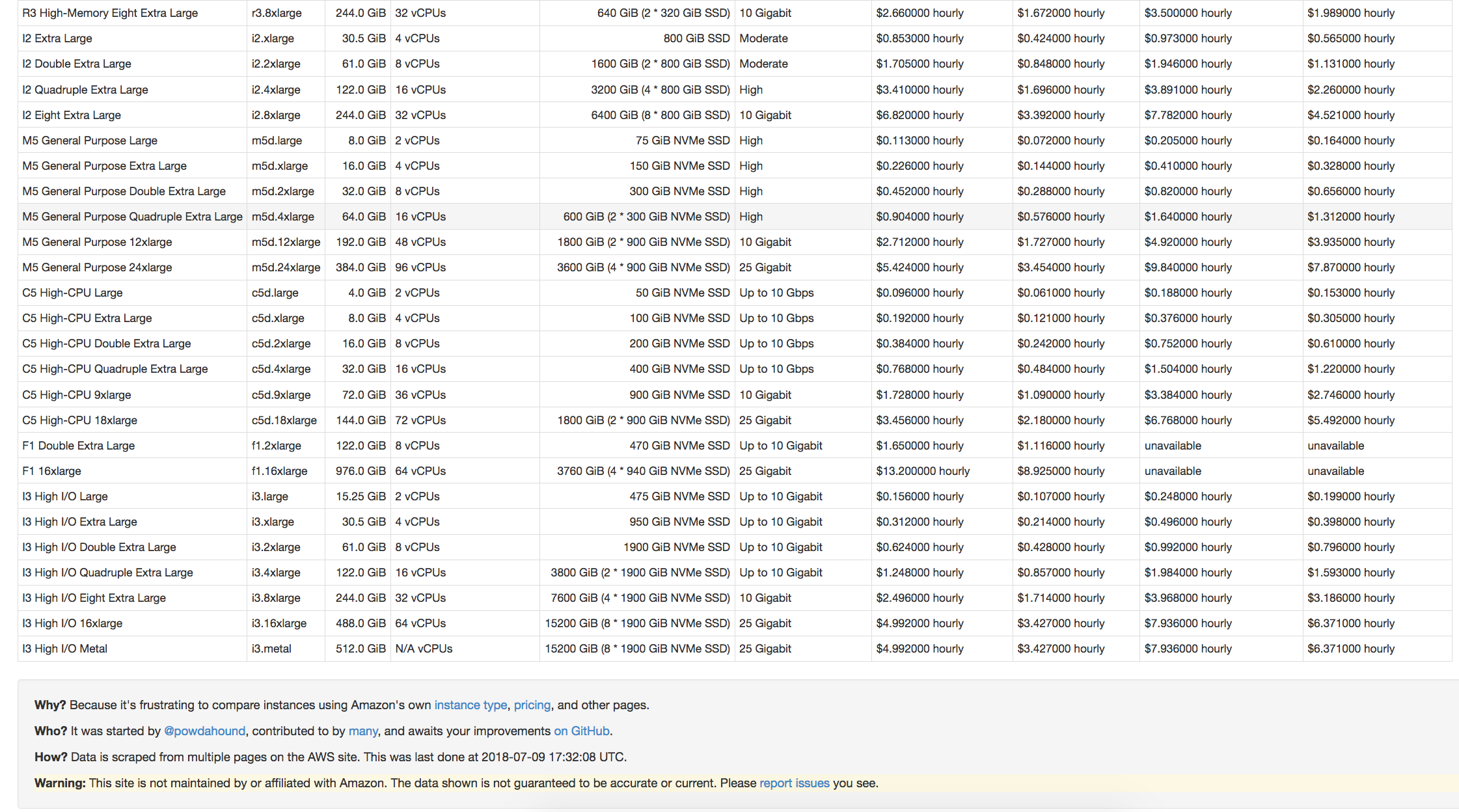ec2instances.info

## Challenge

Reap the benefits of AWS from the comfort of our Python env

Have an adventure without leaving The ShireBy Tom Hall [CC BY 2.0 ], via Wikimedia Commons## Previous attempts

Other platforms have sought to lower the AWS entry barrier

• PiCloud (2010), acquired by Dropbox in 2013
• pyWren (2017), built on AWS Lambda
• 5 minute execution time
• 1.5 GB of RAM
• 512 MB local storage
• no root access

## AWS BatchPros:

• Abstracts away infrastructure details
• Dynamically provisions AWS resources based on requirements of user-submitted jobs
• Allows scientists to run 100,000+ batch jobs

Cons:

• AWS Web Console resists automation
• Requires learning new terminology
• Does not easily facilitate reproducibility

## API

• an abstraction on top of Executor from Python's concurrent futures
• pedagogical example: estimating using Monte Carlo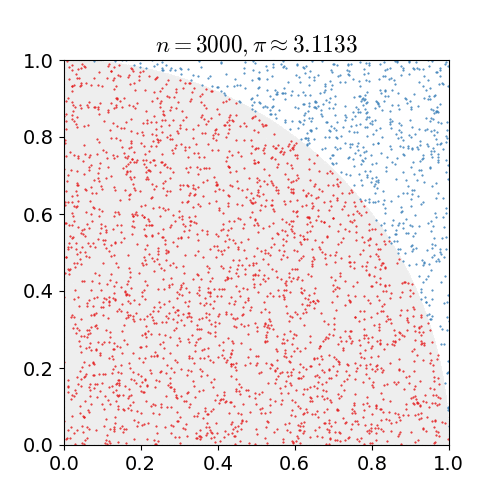By nicoguaro [CC BY 3.0], from Wikimedia Commons

Define the user defined function (UDF).


import cloudknot as ck

def monte_pi_count(n):
import numpy as np
x = np.random.rand(n)
y = np.random.rand(n)
return np.count_nonzero(x * x + y * y <= 1.0)



N.B. we import prerequisites inside the UDF.


import cloudknot as ck

def monte_pi_count(n):
import numpy as np
x = np.random.rand(n)
y = np.random.rand(n)
return np.count_nonzero(x * x + y * y <= 1.0)



Instantiate a Knot, creating resources on AWS.


import cloudknot as ck

def monte_pi_count(n):
import numpy as np
x = np.random.rand(n)
y = np.random.rand(n)
return np.count_nonzero(x * x + y * y <= 1.0)

knot = ck.Knot(name='pi-calc', func=monte_pi_count)



Submit jobs with the map() method.


import cloudknot as ck

def monte_pi_count(n):
import numpy as np
x = np.random.rand(n)
y = np.random.rand(n)
return np.count_nonzero(x * x + y * y <= 1.0)

knot = ck.Knot(name='pi-calc', func=monte_pi_count)

n_jobs, n_samples = 1000, 100000000
import numpy as np
args = np.ones(n_jobs, dtype=np.int32) * n_samples
future = knot.map(args)



Summarize the status of submitted jobs.


import cloudknot as ck

def monte_pi_count(n):
import numpy as np
x = np.random.rand(n)
y = np.random.rand(n)
return np.count_nonzero(x * x + y * y <= 1.0)

knot = ck.Knot(name='pi-calc', func=monte_pi_count)

n_jobs, n_samples = 1000, 100000000
import numpy as np
args = np.ones(n_jobs, dtype=np.int32) * n_samples
future = knot.map(args)

knot.view_jobs()
[out]: Job ID          Name           Status
----------------------------------------
fcd2a14b...     pi-calc-0      PENDING



Query the result status.


import cloudknot as ck

def monte_pi_count(n):
import numpy as np
x = np.random.rand(n)
y = np.random.rand(n)
return np.count_nonzero(x * x + y * y <= 1.0)

knot = ck.Knot(name='pi-calc', func=monte_pi_count)

n_jobs, n_samples = 1000, 100000000
import numpy as np
args = np.ones(n_jobs, dtype=np.int32) * n_samples
future = knot.map(args)

knot.view_jobs()

done_yet = future.done()



Retrieve the result.


import cloudknot as ck

def monte_pi_count(n):
import numpy as np
x = np.random.rand(n)
y = np.random.rand(n)
return np.count_nonzero(x * x + y * y <= 1.0)

knot = ck.Knot(name='pi-calc', func=monte_pi_count)

n_jobs, n_samples = 1000, 100000000
import numpy as np
args = np.ones(n_jobs, dtype=np.int32) * n_samples
future = knot.map(args)

knot.view_jobs()

done_yet = future.done()
res = future.result()



Or retrieve previously submitted results.


import cloudknot as ck

def monte_pi_count(n):
import numpy as np
x = np.random.rand(n)
y = np.random.rand(n)
return np.count_nonzero(x * x + y * y <= 1.0)

knot = ck.Knot(name='pi-calc', func=monte_pi_count)

n_jobs, n_samples = 1000, 100000000
import numpy as np
args = np.ones(n_jobs, dtype=np.int32) * n_samples
future = knot.map(args)

knot.view_jobs()

done_yet = future.done()
res = future.result()
res = knot.jobs[-1].result() # Equivalent to future.result()



Or add a callback to the final result


import cloudknot as ck

def monte_pi_count(n):
import numpy as np
x = np.random.rand(n)
y = np.random.rand(n)
return np.count_nonzero(x * x + y * y <= 1.0)

knot = ck.Knot(name='pi-calc', func=monte_pi_count)

n_jobs, n_samples = 1000, 100000000
import numpy as np
args = np.ones(n_jobs, dtype=np.int32) * n_samples
future = knot.map(args)

knot.view_jobs()

done_yet = future.done()
res = future.result()
res = knot.jobs[-1].result()  # Equivalent to future.result()

PI = 0.0
def pi_from_future(future):
global PI
PI = 4.0 * np.sum(future.result()) / (n_samples * n_jobs)



## Workflow without Cloudknot

• Build a Docker image (local machine)
• Create an Amazon ECR repository for the image (web)
• Push the build image to ECR (local machine)
• Create IAM Roles, compute environment, job queue (web)
• Create a job definition that uses the built image (web)
• Submit jobs (web)

## Single Program


import cloudknot as ck

def awesome_func(...):
...

knot = ck.Knot(func=awesome_func)## Multiple Data


import cloudknot as ck

def awesome_func(...):
...

knot = ck.Knot(func=awesome_func)

...

future = knot.map(args)## Solving differential equations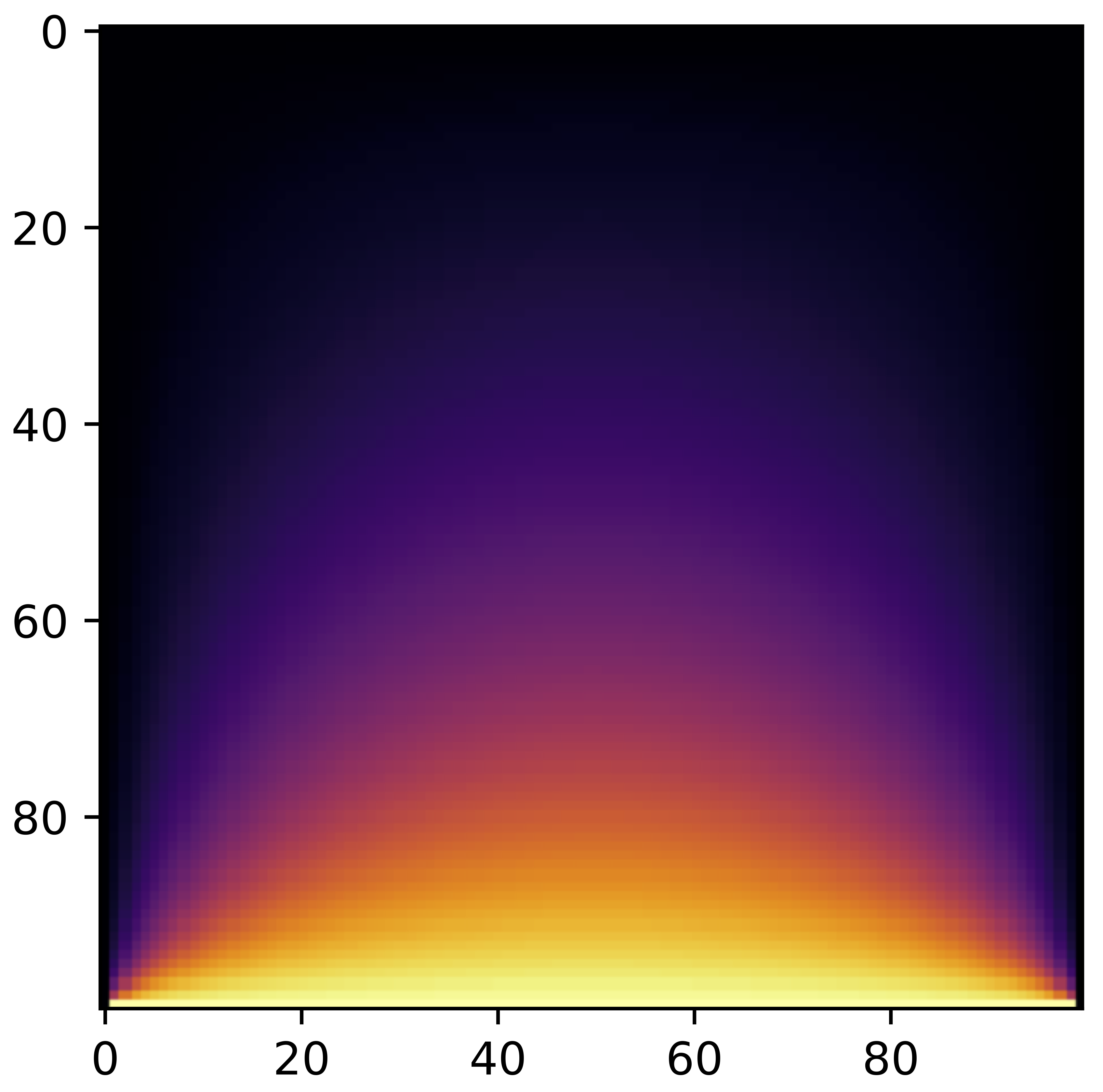Solve

with some boundary conditions.

## Solving differential equations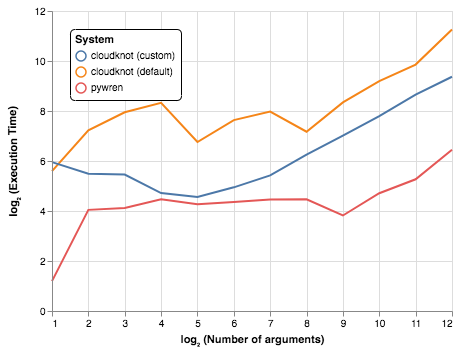Increase number of constraints.

## Solving differential equations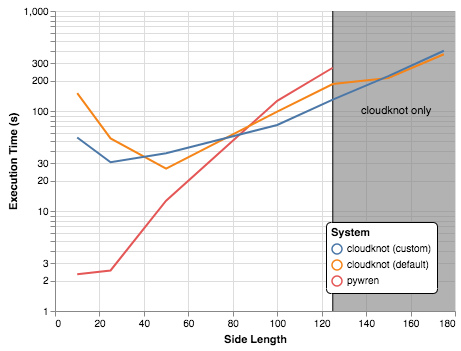Increase system size.

## Solving differential equations

### Takeaway

• If UDF fits within AWS Lambda limitations, use Pywren.
• If not, Cloudknot is here for you.

## Analysis of MRI dataBy everyone's idle (originally posted to Flickr as A brain - I has it) [CC BY-SA 2.0 ], via Wikimedia Commons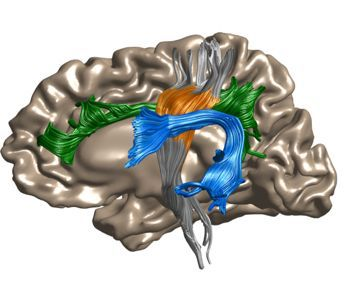Yeatman, et al.; Nature Communications 9, 940 (2018)

## Analysis of MRI data

Compare to Dask, Myria, Spark using previous benchmark study.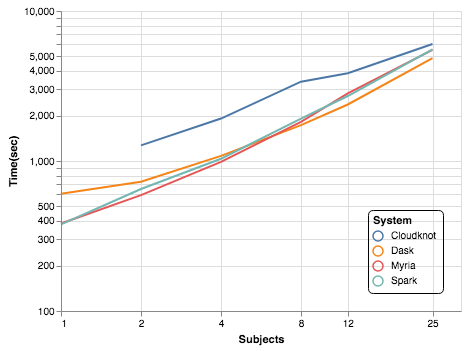## Analysis of MRI data

### Takeaway

• Previous MRI benchmark was performed by a team of 4 graduate students and postdocs over 6 months.
• Cloudknot implementation took Ariel one day
• For 25 subjects, Cloudknot was 10-25% slower
• Cloudknot favors workloads where development time is more important than execution time

## Analysis of microscopy data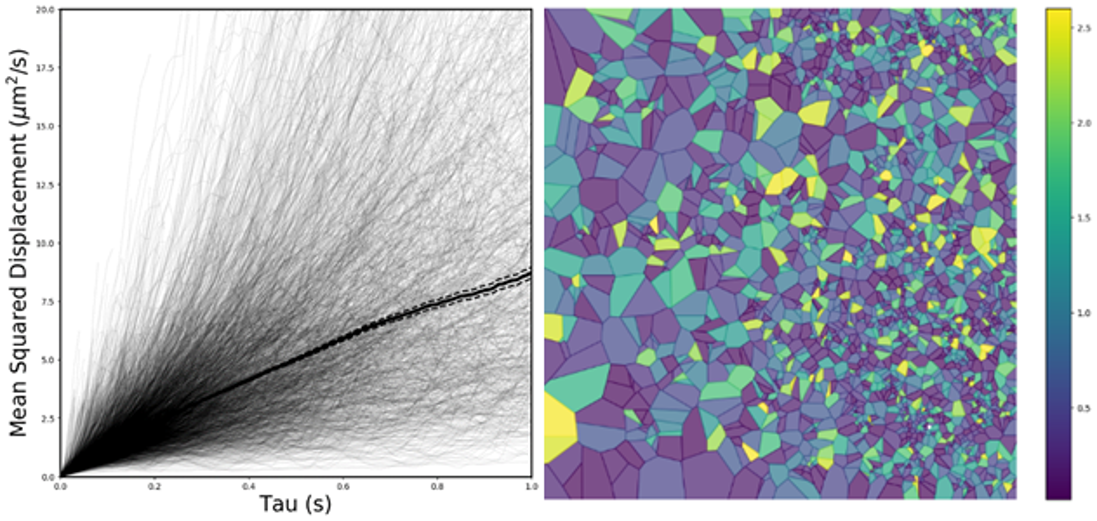## Analysis of microscopy data

• Complicated software dependencies
• ImageJ and TrackMate
• Written in Java, scripted in Jython
• Installation can be managed in a Docker image
• Large datasets, measured in TB
• Managed using an AMI that includes a larger volume
• Default Batch AMI volumed limited to 30 GB
• Takeaways
• Longer development time due to custom AMI and Docker image
• This lab has completely transitioned from using bespoke cluster to AWS.
• Enables capability computing (rather than capacity computing)

## Conclusion

• Cloudknot favors workloads where development time matters more than execution time.
• For many data science problems, this is an acceptable trade.
• Simplified API makes cloud computing more accessible.
1. import cloudknot
2. cloudknot.Knot()
3. map() method

Github repo: https://github.com/richford/cloudknot

Documentation: https://richford.github.io/cloudknot/index.html

We welcome issues and contributions!